International
Tables for
Crystallography
Volume F
Crystallography of biological macromolecules
Edited by M. G. Rossmann and E. Arnold

International Tables for Crystallography (2006). Vol. F, ch. 13.3, pp. 275-276   | 1 | 2 |

Section 13.3.2. R-factor and correlation-coefficient translation functions

L. Tonga*

aDepartment of Biological Sciences, Columbia University, New York, NY 10027, USA
Correspondence e-mail: tong@como.bio.columbia.edu

13.3.2. R-factor and correlation-coefficient translation functions

| top | pdf |

The crystallographic R factor is often used as an indicator in translation searches. It is a measure of the percentage difference between the observed (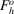) and the calculated (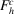) structure-factor amplitudes,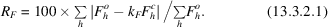A similar R factor can be defined based on the square of the structure-factor amplitudes, i.e. an R factor based on intensity,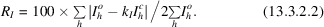A factor of 2 is introduced in equation (13.3.2.2) to makevalues fall in the same range as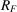. In equations (13.3.2.1) and (13.3.2.2)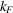and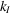are scale factors that bring the observed and the calculated structure factors to the same level. These scale factors are generally calculated in shells of equal reciprocal volume, which can compensate for differences in the displacement factors between the observed and the calculated structure-factor amplitudes.

In addition to the R factor, the correlation coefficient between the observed and the calculated structure factors is also used in translation searches. Like the R factors, correlation coefficients can be defined based on the amplitude or the intensity of the reflections, and that based on the amplitude is shown below.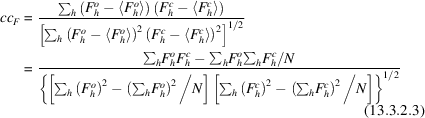In (13.3.2.3), N is the number of reflections that are used in the calculation and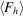denotes the average structure-factor amplitude over the reflections. Unlike the R factors, the correlation coefficients do not depend on the overall scale factor between the observed and the calculated structure factors. However, they can be affected by large differences in the overall displacement factors between the observed and the calculated structure factors.

In order to evaluate R factors and correlation coefficients for a translation search, structure factors need to be calculated for the search model, with a given orientation, at different positions in the unit cell. For this special case, where only the positional parameters of the search model are varied, the calculation of the structure factors can be simplified (Nixon & North, 1976; Rae, 1977). The structure-factor equation can be written as a double summation – first over the atoms in one asymmetric unit of the unit cell and then over all the asymmetric units,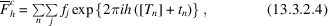where j goes over all the atoms in the asymmetric unit and n goes over all the crystallographic asymmetric units. The nth crystallographic symmetry operator is given by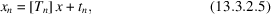where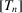is the rotational component and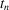is the translational component of the symmetry operator.

For simplicity, first consider the case where there is only one molecule in the asymmetric unit. In the translation search, the model will be placed at different positions in the unit cell,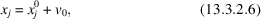where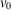is a translation vector which is applied to move the model from its starting position (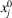). Substitutingin (13.3.2.6) into (13.3.2.4) gives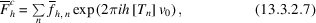where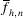is the structure factor calculated based only on the nth symmetry-related molecule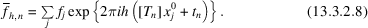It can be calculated by placing the search model in a P1 unit cell having the same cell dimensions as the unknown-crystal unit cell. The structure factors calculated for this P1 cell are related toby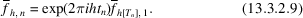Therefore, the summation over the atoms in the structure-factor calculation, a rather time-consuming process, needs to be performed only once, for the search model at the starting position. Subsequent structure-factor calculations after translation of the model are no longer dependent on the number of atoms present in the unit cell [equation (13.3.2.7)]. The starting position is usually chosen such that the centre of the search model is at (0, 0, 0). Then the vector that is determined from the translation searches will define the centre of the model in the unit cell.

Equation (13.3.2.7) can be generalized to allow for the presence of other molecules that are to remain stationary during the translation search: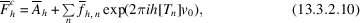where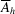is the contribution from the stationary molecules. This formulation is useful if there is more than one molecule in the asymmetric unit. The position of one of the molecules can be determined first, and the model is then included as a stationary molecule for the position search of the next molecule.

Evaluation of the R factor and the correlation coefficient [equations (13.3.2.1) and (13.3.2.3)] in a translation search is generally rather slow. A method has been developed to calculate the correlation coefficient by the fast Fourier transform (FFT) technique, which involves reciprocal vectors up to four times the resolution of the reflection data (Navaza & Vernoslova, 1995).

Equation (13.3.2.7) can also be generalized to allow for the presence of two (or more) search models that are to move independently of each other during the translation search. However, this will generally lead to a six- (or more) dimensional problem and is extremely expensive in computation time. With recent improvements in computer technology (especially parallel processing), it might be feasible to carry out such searches in special cases. However, this aspect of translation functions will not be discussed further here.

References

Navaza, J. & Vernoslova, E. (1995). On the fast translation functions for molecular replacement. Acta Cryst. A51, 445–449.Google Scholar
Nixon, P. E. & North, A. C. T. (1976). Crystallographic relationship between human and hen-egg lysozymes. I. Methods for the establishment of molecular orientational and positional parameters. Acta Cryst. A32, 320–325.Google Scholar
Rae, A. D. (1977). The use of structure factors to find the origin of an oriented molecular fragment. Acta Cryst. A33, 423–425.Google Scholar